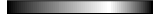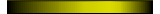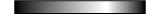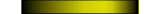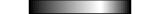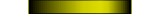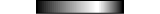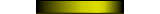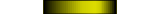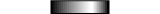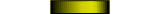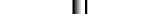# Tower of Hanoi algorithm

1.- Introduction

2.- Presentation of main ideas and code

3.- Play the Puzzle!!

### 1.- Introduction to the algorithm of the Towers of Hanoi in Matlab

 Would you like to get the Tower of Hanoi algorithm? For the moment, see how you do in this wonderful game of skill and logic... The Towers of Hanoi is a mathematical game or puzzle. It consists of three rods, and a number of disks of different sizes which can slide onto any rod.

The puzzle starts with the disks in a neat stack in ascending order of size on one rod, the smallest at the top, and then making a conical shape.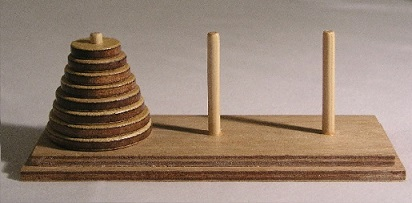Try to move all the disks onto another pole. Thing is, you can only
move one disk at a time and you must follow size order (a bigger disk
can't go on a smaller disk). Good luck!

### 2.- Solution using recursivity

The following presentation gives you a very good idea of what recursivity is.
You can examine the full code in Matlab after the presentation.

This is the main function (the one that is going to call itself many times).

function m(n, init, temp, fin)
if n == 1
disp([init ' to ' fin])

else
% move n-1 disks from A to B, with C as temp
m(n-1, init, fin, temp);

% move disk from A to C, with B as temp
m(1, init, temp, fin);

% move n-1 disks from B to C, with A as temp
m(n-1, temp, init, fin);
end

You can call it providing the number of disks and the names of the towers.

n = 3;
m(n, ' A', ' B', ' C')

This is the complete result of the moves for 3 disks...

A to C
A to B
C to B
A to C
B to A
B to C
A to C

### 3.- Play the Game!

Free JavaScripts provided
by The JavaScript Source